## Biomedical Research

All submissions of the EM system will be redirected to Online Manuscript Submission System. Authors are requested to submit articles directly to Online Manuscript Submission System of respective journal.

- Biomedical Research (2016) Volume 27, Issue 1

## Performance evaluation of DWT, SWT and NSCT for fusion of PET and CT Images using different fusion rules.

 KP Indira1*, R Rani Hemamalini2, NM Nandhitha3 1Sathyabama University, Chennai. 2St.Peter’s College of Engineering, Chennai. 3Deptartment of ETCE, Sathyabama University, Chennai. Corresponding Author: KP Indira Sathyabama University, Chennai. Accepted: November 26, 2015
Visit for more related articles at Biomedical Research

### Abstract

Medical image fusion is the method of combining or merging complementary information from two or more source images into a single image to enhance the diagnostic capability. In this work six different fusion rules are performed for Discrete Wavelet Transform (DWT), Stationary Wavelet Transform (SWT) and Non Subsampled Contourlet Transform (NSCT) using eight sets of real time medical images. For fusing low frequency coefficients, average and choose max fusion rules are used. For the fusion of high frequency coefficients choose max, gradient and contrast fusion rules are used based on pixel based rule. The proposed technique is performed using eight groups of Positron Emission Tomography (PET), Computed Tomography (CT) medical images. The performance of DWT, SWT and NSCT are compared with four different quality metrics. From experimental output average, gradient fusion rule outperforms other fusion rules from both subjective and objective estimation. It is also observed that the time taken for the execution of images is more for Stationary Wavelet Transform (SWT) than Discrete Wavelet Transform (DWT) and Non Subsampled Contourlet Transform (NSCT).

### Keywords

Discrete Wavelet Transform (DWT), Stationary Wavelet Transform (SWT), Non Subsampled Contourlet Transform (NSCT), Average, Choose max, Contrast, Gradient fusion Rules

### Introduction

Image fusion refers to the practice of amalgamating two or more images into a composite image that assimilates the information comprised within the individual image without any artifacts or noise. Multi-modal medical image fusion is an easy entrance for physicians to recognize the lesion to analyze images of different modalities . This has been emerging as a new and talented area of research due to the increasing demands in clinical applications. The area of biomedical image processing is a rapidly rising area of research from last two decades . Medical imaging is sub divided into functional and structural information where magnetic resonance imaging (MRI) and computed tomography (CT) afford high-resolution images by means of structural and anatomical information whereas positron emission tomography (PET) and single-photon emission computed tomography (SPECT) images afford functional information with low spatial resolution. Hence the goal is to reckon the content at each pixel location in the input images and preserve the information from that image which best represents the true scene significant content or enhances the effectiveness of the fused image for a precise application.
Here a novel method of six different fusion rules is used for SWT, DWT and NSCT. These fusion rules are applied for eight sets of PET, CT images. Choose max, average fusion rules are applied for low frequency coefficients and for high frequency coefficients choose max, gradient and contrast fusion rules are applied and tested both qualitatively and quantitatively. Section 2 briefly explains related work, proposed methodology is given in Section 3, and fusion results are given in Section 4, quantitative analysis of different fusion rules are given in section 5, global comparison between different fusion rules are given in section 6 and conclusion in Section 7.

### Related Work

Rajiv Singh, Ashish Khare et al., proposed complex wavelet transform which fuses coefficient of input source images using maximum selection rule  .These results are compared with LWT, MWT, SWT and also with CT, NSCT, DTCWT and PCA methods. For fusion of images maximum selection rule is applied from level 2 to 8 for three different sets of multimodal medical images. Further it is concluded that the results obtained proves that the quality of fused image increases, as the level increases. Andreas Ellmauthaler et al., proposed a fusion scheme based on Undecimated wavelet transform . This splits the image decomposition procedure into two sequential filtering operations by spectral factorization of analysis filters. Here fusion takes place subsequent to convolution with the first filter pair. Best results are obtained by applying UWT calculation of low-frequency coefficients and the outcome are compared with wavelets . The coefficients of two different types of images through beyond wavelet transform are obtained and then the low-frequency and high frequency coefficients are selected by maximum local energy and sum modified Laplacian method. Ultimately, the output image is procured by performing an inverse beyond wavelet transform. The results show that the maximum local energy is a new approach for obtaining image fusion with adequate performance. Yi Li, Guanzhong Liu proposed cooperative fusion mode, where it is considered the activity levels of SWT and NSCT at the same time . Initially, every source image is decomposed by SWT and NSCT. Later fused coefficients are attained by combining the NSCT coefficients, by taking into account both the SWT coefficients and NSCT coefficients. Manoj D. Chaudhary, Abhay B. Upadhyay et al., proposed a method where the images are extracted using SWT initially and then global textural features are extracted by gray level co-occurrence matrix . Different DWT, SWT based image fusion methods are discussed in [8-14].

### Proposed Methodology

As fusion rules play a significant role in image fusion, to fuse images after decomposition average, choose max rules are applied for low frequency and for high frequencies contrast, gradient and choose max rules are utilized for DWT /SWT/NSCT. The simple block diagram representation is specified below in Figure 1.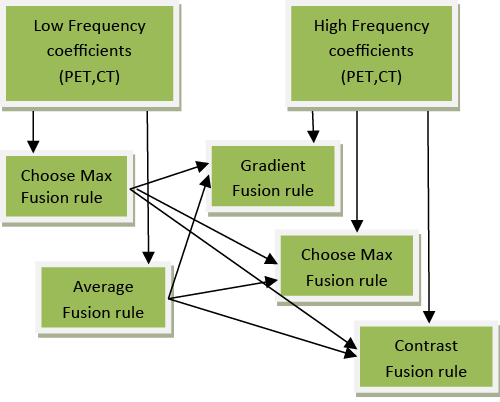Figure 1: Different fusion rules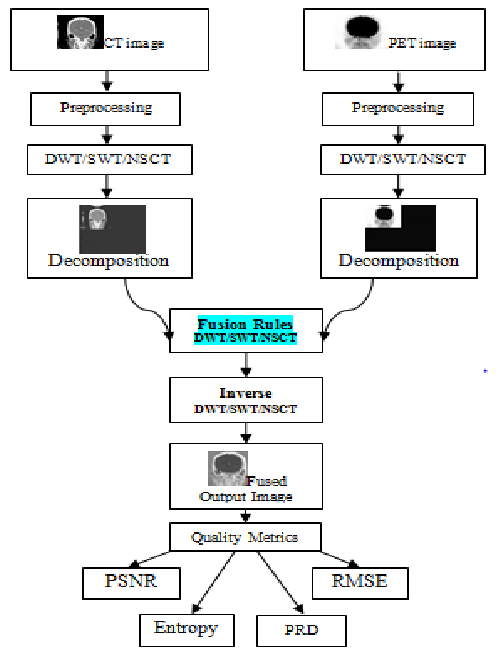Figure 2: Proposed image fusion algorithm
The block diagram illustration of the proposed algorithm is specified below in Figure 2. The initial step is to acquire PET and CT images as input. In image preprocessing after retrieving the input images, to speed up execution time, image resizing is performed followed by RGB to gray conversion.
Next step is to decompose the images into LL, LH, HL and HH frequency coefficients using DWT/SWT/NSCT. For low frequency coefficients choose max, average rules are applied whereas choose max, gradient and contrast fusion rules are used for high frequency coefficients. Different fusion rules are implemented for DWT/SWT/NSCT. To reconstruct the original images inverse transform is applied and to validate the results different performance metrics are used.

#### Discrete wavelet transform (DWT)

The discrete wavelet transform (DWT) is a direct transformation that works on an information vector whose length is a whole number power of two, changing it into a numerically diverse vector of the same length. This isolates information into distinctive frequency components, and studies every segment with resolution coordinated to its scale . DWT of an image delivers a non-redundant image representation, which gives better spatial and spectral localization compared to existing multiscale representations. It is computed with a cascade of filters followed by a factor 2 sub sampling and the principle highlight of DWT is multi scale representation. By utilizing the wavelets, given functions can be analyzed at different levels of resolution. DWT decomposition utilizes a course of low pass and high-pass channels and a subsampling operation. The yields from 2D-DWT are four images having size equal to half the size of input image. So from first input image HHa, HLa, LHa, LLa images are obtained and from second input image HHb, HLb, LHb, LLb images are obtained. Here LL image contains the approximation coefficients. LH image contains the horizontal detail coefficients. HL image contains the vertical detail coefficients and HH contains the diagonal detail coefficients. One of the significant disadvantages of wavelet transform is their absence of translation invariance .

#### Stationary wavelet transform (SWT)

The stationary wavelet transform (SWT) is an expansion of standard discrete wavelet transform (DWT) that utilizes high and low pass channels. SWT apply high and low pass channels to the information at every level and at next stage it produces two sequences. The two new successions will have same length as that of first grouping. In SWT, rather than annihilation the channels at every level is altered by cushioning them with zeroes. Stationary Wavelet Transform is computationally more complex. The Discrete Wavelet Transform is a time variant transform. The best approach to restore the interpretation invariance is to average some slightly distinctive DWT, called undecimated DWT to characterize the stationary wavelet transform (SWT) . SWT does this by suppressing the down-sampling step of the DWT and instead up-sampling the filters by padding with zeros between the filter coefficients. After decomposition, four images are generally furnished (one approximation and three detail coefficients) which are at half the resolution of the original image in DWT, whereas in SWT the approximation and detail coefficients will have the same size as the input images. SWT is like discrete wavelet transform (DWT), however the main procedure of down-sampling is stifled which implies that SWT is shift invariant. It applies the DWT and excludes both downsampling in the forward and up-sampling in the reverse direction. More precisely, it executes the transform at each point of the image and saves the detail coefficients and uses the low frequency information at each level.

#### Non subsampled contourlet transform (NSCT)

Wavelet transform has been considered as a ideal strategy for image fusion . Despite the fact that DWT is most normally used, it suffers from shift variance issue. To overcome the above issue SWT was proposed. Although SWT is shift invariant technique, it performs better at segregated discontinuities, yet not at edges and textured locals. To conquer the above drawbacks and to hold the directional and multi scale properties of the transform non subsampled contourlet transform (NSCT) has been proposed which decomposes the images in the form of contour segments. Therefore, it can capture geometrical structure of an image in a more efficient manner than existing wavelet techniques. NSCT is an amalgamation of both non subsampled pyramid and non-subsampled directional filter bank. Also this is a geometric evaluation technique that utilizes the geometric regularity which is present in the individual input images and furnishes an output image with better localization, multi-direction and shift invariance.

### Fusion Rules

Selection of fusion rules plays a significant role in image fusion. Most information of the source images is kept in the low-frequency band as it is a smoothed and subsampled version of original input image . Higher value of wavelet coefficients carries salient information about images such as corners, edges and hence maximum selection rule, gradient and contrast fusion rule has been chosen for fusion .

#### Maximum or choose max fusion rule

Higher value of wavelet coefficients contains most important information about images such as edges, and corners . Therefore, in maximum selection rule for fusion, smaller magnitude complex wavelet coefficients are replaced by means of higher magnitude complex wavelet coefficients. For every corresponding pixel in input images, the pixel with the maximum intensity is chosen and used as the resultant pixel of the fused image. The major steps of the proposed algorithm are summarized as follows:
If,
LL1(i,j) > LL2(i,j)
Lout(i,j) = LL1(i,j);
else
Lout(i,j) = LL2(i,j);
Where, LL=indicates low frequency coefficients, Lout=indicates output image value, LL1=indicates coefficients of CT image and LL2=indicates coefficients of PET image.

#### Average fusion rule

This method is a simple one where fusion is achieved by calculating average of corresponding pixel in each input image.
Low frequency components are fused by averaging method.
Mean = (LL Part of PET Image + LL Part of CT Image)/2.

#### Gradient rule

The term image gradient is a directional change in the intensity or color of an image that may be used to extract information. This considerably reduces the amount of distortion artifacts and contrast information loss that is observed in fused output images obtained from general multiresolution fusion schemes . This is because; fusion in the gradient map domain considerably improves the reliability of information fusion processes and the feature selection. Gradient represents the steepness and direction of that slope. The appropriate high frequency sub bands are chosen (LH, HL and HL) to find out the gradient value. These values of two input images are compared and the better values are taken as the output and given by,
dx = 1;
dy = 1;
[dzdx1,dzdy1] = gradient (LH1,dx,dy);
gm1 = sqrt ((dzdx1 .^ 2 + dzdy1 .^2));
where,
dx- Slope along horizontal direction.
dy- Slope along vertical direction.
dz- Slope along diagonal direction.

#### Contrast rule

Contrast measures the difference of the intensity value at some pixel from the neighboring pixels as human visual system is very sensitive to the intensity contrast rather than the intensity value itself. Initially the mean value for low frequency part is calculated. Then maximum values for the LL, HL, LH and HH part are calculated.
Contrast value = Mean/Maximum value of the visible sub band
Contrast values of two input images are compared and then mean and maximum of the respective sub bands are calculated as below,
AL_M = mean (mean (LL1 (i-1:i+1,j-1:j+1)));
AL_M = mean (mean (LL1 (i-1:i+1,j-1:j+1)));
AL_H = max (max (LH1(i-1:i+1,j-1:j+1)));
AL_V = max (max (HL1(i-1:i+1,j-1:j+1)));
AL_D = max (max (HH1(i-1:i+1,j-1:j+1)));
Con_A_H (i-1,j-1) = AL_H/AL_M;
Con_A_V (i-1,j-1) = AL_V/AL_M;
Con_A_D (i-1,j-1) = AL_D/AL_M;

### Results and Discussion

It is essential to assess the fusion action from both subjective and objective image quality feature measurement. Here the performance of the proposed work is compared with eight sets of real time medical images obtained from Bharat Scans. For DWT, SWT and NSCT six sets of fusion rules are applied for eight sets of PET, CT medical images. For the fusion of low frequency coefficients choose max and average fusion rules are applied whereas choose max, gradient and contrast fusion rules are used for high frequency coefficients. The numerical values for the qualitative measurements are given below followed by quantitative analysis. In Figure 3, column A1 represents Computed Tomography (CT) and A2 represents Positron Emission Tomography (PET) images. The results of the corresponding outputs of CT, PET images are given as output images from A3-A20------ H3-H20. In this, Avg. indicates average, Con. denotes contrast, Gra. denotes gradient and Max. denotes choose max fusion rule. Figure 4

### Global Comparison

Quality assessment of the fused image is complicated in general, as the ideal fused image is often associated by specific tasks. Also subjective methods are complicated to perform as they are based on psycho-visual testing. These are also expensive in terms of time and equipment required. Furthermore, there is slight difference between fusion results and hence subjective means are difficult to evaluate the correct fusion results. A lot of objective evaluation methods have been developed for these reasons and four of them are given below.

#### Peak signal to noise ratio (PSNR)

As higher values of PSNR gives better results, for DWT and SWT the average, gradient fusion rule gives good results for all eight image sets. On comparing PSNR values of NSCT with different fusion rules average, gradient fusion rule gives better results for image sets 2, 3, 4, 5, 6, 7 and 8 while maximum, gradient fusion rule gives better result for image set 1.

#### Root mean square error (RMSE)

On comparing RMSE values of DWT with different fusion rules average, gradient fusion rule gives better results for image sets 2, 3, 4, 5, 7 and 8. Maximum, contrast fusion rule gives better result for image set 1while average, maximum fusion rule gives better result for image set 6. For SWT with different fusion rules average, gradient fusion rule gives better results for all image sets. On the other hand for NSCT average, gradient fusion rule gives better results for image sets 2, 3, 4, 5, 6, 7 and 8 while maximum, contrast rule gives better result for image set 1 as lower values of RMSE gives better results.

#### Entropy

Entropy of an image designates the information content of the merged image and hence its value must be high. On comparing entropy values of DWT with different fusion rules average, gradient fusion rule gives better results for image sets 1,2,4,5,6,7 and 8 while maximum, contrast fusion rule gives better result for image set 3. For SWT with different fusion rules average, gradient fusion rule gives better results for images 1, 2, 3, 4, 5,6and 8 and average, maximum fusion rule gives better result for image set 7. On comparing entropy values of NSCT average, gradient fusion rule gives better results for images 2, 3, 4, 5, 6, 7 and 8 and average, contrast fusion rule gives better result for image set 1.

#### Percentage Residual Difference (PRD)

While comparing PRD values of DWT with different fusion rules average, gradient fusion rule gives better results for all image sets. On comparing PRD values of SWT average, gradient fusion rule gives better results for images 1,2,3,4,5,6 and 8 and average, contrast fusion rule gives better results for image set 7 . For NSCT average, gradient fusion rule gives better results for image sets 2, 3, 4, 5, 6,7 and 8 while maximum, contrast fusion rule gives better result for image set 1.Figure 3: Results for different fusion rules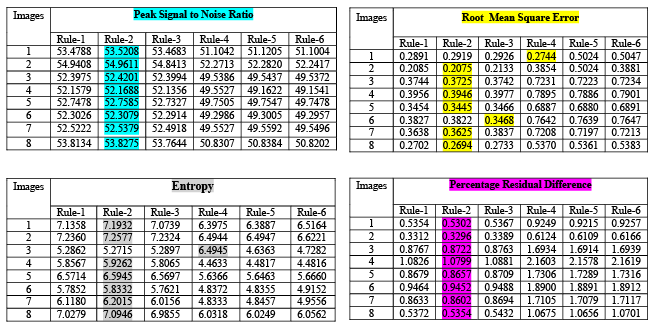Figure 4A: Quantitative analysis of Discrete Wavelet Transform (DWT)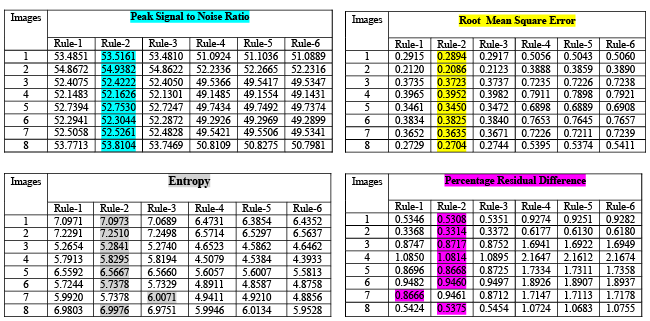Figure 4B: Quantitative analysis of Stationary Wavelet Transform (SWT)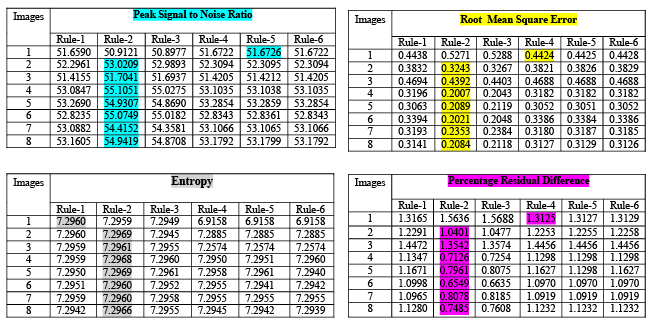Figure 4C: Quantitative analysis of Non Subsampled Contourlet Transform (NSCT)

### Conclusion

A novel pixel based image fusion method using using six different fusion rules are proposed in this paper and the results are emphasized in section 4 for discrete wavelet transform, stationary wavelet transform and non subsampled contourlet transform. From the observation of the results it is clear that average fusion rule for low frequency coefficient and gradient fusion rule for high frequency coefficient provides better results than other fusion rules for all discrete wavelet transform (DWT), stationary wavelet transform (SWT) and Non subsampled contourlet transform (NSCT). Pixel level fusion is suffered by blurring effect that directly affects the contrast of the image in maximum selection rule, compared to average fusion rule. Hence for low frequency coefficients average fusion rule is more suitable than the other. Gradient fusion considerably minimizes the loss of contrast information and amount of distortion artifacts in fused images. Also this is because fusion in the gradient map domain significantly improves reliability of information fusion processes and the feature selection. Hence for high frequency gradient based fusion rule is more suitable than other two. Also the time taken for the execution of SWT is more than DWT and NSCT. Hence from the observation it is concluded that average and gradient based fusion rules works better for bio medical images than other fusion rules.

### References

1. Wang L, Li B, Tian LF. Multi-modal medical image fusion using the inter-scale and intra-scale dependencies between image shift-invariant shearlet coefficients 2014; 19: 20-28.
2. Daneshvar S,Ghassemian H. MRI and PET image fusion by combining IHS and retina-inspired models 2010; 11: 114-123.
3. Singh R, Khare A. Fusion of multimodal medical images using Daubechies complex wavelet transform ? A multiresolution approach 2014; 19: 49-60.
4. Ellmauthaler A, Carla L. Pagliari , Da Silva AB. Multiscale Image Fusion Using the Undecimated Wavelet Transform With Spectral Factorization andNonorthogonal Filter Banks. IEEE Transactions on image processing 2013;22: 1005-1017.
5. Huimin Lu, Lifeng Zhang, Seiichi Serikawa. Maximum local energy: An effective approach for multisensor image fusion in beyond wavelet transform domain 2012; 64: 996-1003.
6. Yi Li, Guanzhong Liu. Cooperative Fusion of Stationary Wavelet Transform and Non-subsampled Contourlet for Multifocus Images 2009;1: 314-317.
7. Chaudhary MD, Upadhyay AB. Fusion of local and global features using Stationary Wavelet Transform for EfficientContent BaseImage Retrieval 2014; 1-6.
8. Huang PW, Chen CI, Li PL. PET and MRI Brain Image Fusion Using Wavelet Transform with Structural Information Adjustment and Spectral Information Patching. 2014; 1-4.
9. Sahoo T, Patnaik S. Cloud Removal from Satellite Images using Auto Associative Neural Network and StationaryWavelet Transform2008; 100-105.
10. Shi H, Fang M. Multi-focus Color Image Fusion Based on SWT and HIS. 2007; 461-465.
11. Chabira B, Skanderi T, AichoucheBelhadjaissa. Unsupervised Change Detection from Multi temporal Multichannel SAR Images based on Stationary Wavelet Transform 2013; 1-4.
12. Zhang X,Zheng Y, Peng Y. Research on Multi-Mode Medical Image Fusion Algorithm Based on Wavelet Transform and the Edge Characteristics of Images 2009; 1-4.
13. Nunez J, Otazu X, Fors O, Prades A. Multiresolution-Based Image Fusion with Additive Wavelet Decomposition. IEEE Transactions on Geoscience and remote sensing 1999; 37: 1204-1211.
14. Kok C, Hui Y, Nguyen T. Medical images pseudo coloring by wavelet fusion. Bridging disciplines for biomedicine. Proceedings of 18th Annual International Conference of the IEEE 1996; 2: 648-649.
15. Kannan K, Perumal SA, Arulmozhi S. Optimal Decomposition Level of Discrete, Stationary and Dual Tree Complex Wavelet Transform for Pixel based Fusion of Multi-focused Images. Serbian Journal of Electrical Engineering2010; 7: 81-93.
16. Simoncelli EP, Freeman WT,Adelson EH, Heeger DJ. Shiftable MultiscaleTransforms. IEEETransactionsonInformation Theory 1992; 38.
17. Pesquet JC, Krim H, Carfatan H.Time-invariant orthonormal wavelet representations. IEEE Trans on Signal Processing1996; 44.
18. Bhatnagar G, WuJQ, Zheng Liu Z. Directive contrast based multimodal dical image fusion in NSCT domain. IEEE Transactina2013; 15: 1014-1024.
19. Yang Y, Park DS, Huang S, Rao N. Medical Image Fusion via an Effective Wavelet-Based Approach. Journal on Advances in Signal Processing 2010.
20. IndiraKP ,Rani Hemamalini R. Impact of co-efficient selection rules on the performance of DWT based fusion on medical images2015; 1-8.
21. Petrovic VS,XydeasCS.Gradient-based multiresolutionimage fusion 2004; 13: 228-237.